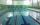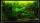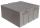# Cuboid - 7th grade (12y) - math problems

A cuboid is a three-dimensional shape with a length, width, and a height. A cuboid is a rectangular Prism. The cuboid shape has six sides called faces. Each face of a cuboid is a rectangle, and all of a cuboid's corners (called vertices) are 90-degree angles. Opposite faces are parallel. A cuboid has the shape of a rectangular box.

#### Number of problems found: 159

• Pool 3How long will fill pool cuboid shape (8m 6m 1.5m) when flows 15 liters/s?
• AquariumCan 30 liters of water fit in a cuboid aquarium with dimensions a = 3dm b = 6dm c = 5dm?
• Surface and volumeFind the surface and volume of a cuboid whose dimensions are 1 m, 50 cm, and 6 dm.
• CardboardHow many m2 of cardboard are needed to make the cuboid with dimensions 40 cm 60 cm and 20 cm?
• Water poolWhat water level is in the pool shaped cuboid with bottom dimensions of 25 m and 10 meters, when is 3750hl water in the pool.
• Edge cFind the edge c of cuboid if an edge a = 20 mm, b = 30 mm and surface area S = 8000 mm2.
• HectolitersHow many hectoliters of water fits into cuboid tank with dimensions of a = 3.5 m b = 2.5 m c = 1.4 m?
• Water reservoirThe water tank has a cuboid with edges a= 1 m, b=2 m , c = 1 m. Calculate how many centimeters of water level falls, if we fill fifteen 12 liters cans.
• TankTo cuboid tank whose bottom has dimensions of 9 m and 15 m were flow 1080 hectoliters of water. This was filled 40% of the tank volume. Calculate the depth of the tank.
• Swimming pool 4The pool shaped cuboid measuring 12.5 m × 640 cm at the bottom is 960hl water. To what height in meters reaches the water level?
• Water tankThe water tank shape cuboid has a width of 3.1 m and length twice larger. How high will reach water if water flows into 13 liters of water per second during 16 minutes?
• Cuboid wallsThe base of the block is a rectangle whose sides have lengths in the ratio 13: 7. Find the volume of the block in liters, if the longer side of the base measures 65 cm and the height of the block is 1.2 m
• IcebergWhat is the surface area of 50 cm iceberg (in the shape of a cuboid) that can carry a man with luggage with a total weight of 120 kg?
• Oak cuboidOak timber is rectangular shaped with dimensions of 2m, 30 cm and 15 cm. It weight is 70 kg. Calculate the weight 1 dm³ of timber.
• Water tankWhat is the height of the cuboid-shaped tank with the bottom dimensions of 80 cm and 50 cm if the 480 liters of water reaches 10 cm below the top?
• CremonsThe freight wagon is shaped like cuboid 21m and 3.5m and 4.2m How many cremons can be loaded if one is a cube with an edge length of 7 cm?
• An exampleAn example is playfully for grade 6 from Math and I don't know how to explain it to my daughter when I don't want to use the calculator to calculate the cube root. Thus: A cuboid was made from a block of 16x18x48 mm of modeline. What will be the edge of t
• Concrete tankHow many m3 of concrete is in the tank shape of a cuboid with dimensions of 2000 cm and 5.6 meters and 70 dm, if the concrete takes up 3/7 of the tank?
• WaterInto a full cylindrical tank high 3 with base radius 2.4 m we insert cuboid with dimensions 1.7 m, 1.7 m, 0.8 m. How many liters of water will come out?
• Cuboid 5Calculate the mass of the cuboid with dimensions of 12 cm; 0.8 dm and 100 mm made from spruce wood (density = 550 kg/m3).

Do you have an interesting mathematical word problem that you can't solve it? Submit a math problem, and we can try to solve it.

We will send a solution to your e-mail address. Solved examples are also published here. Please enter the e-mail correctly and check whether you don't have a full mailbox.

Please do not submit problems from current active competitions such as Mathematical Olympiad, correspondence seminars etc...